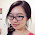### leetcode Question 62: Palindrome Number

Palindrome Number:
Determine whether an integer is a palindrome. Do this without extra space.
Analysis:
The question requires no extra space, so we cannot convert the number to string and check the end and front. But the idea is still check the top and last digit. The last digit is easily achieved by x%10(x mod 10).
To get the next last digit, x=x/10; x%10;  On the other hand, how to get first digit? Similarly, keep x = x/10,  until x<10.  So, if we can get the first digit and the last digit, then we compare, if not equal return false, if equal we search the next two digits. Recursion seems a good idea to solve the problem. 2 same number as the parameter is set to get the first and last digit respectively. So we first recursively get the first digit, then compare to the last digit, if ok, what we is only deal with the 2nd number in order to get the next last digit (by x=x/10). Because the recursion property, when this level is over, return to the earlier level, the last digit of the  1st number is the next highest digit, so we only need to get mod when comparing.

e.g.

121 121
12   121
1     121
0     121
meet 0 return
1     121  (check if 1==121%10)
12   12    (check if 12%10 == 12%10)
12   1     (12/10)
121  1    (check 121%10 == 1%10)
OK!

Code:

class Solution {
public:

bool check(int x, int &y){
if (x==0) {return true;}
if (check(x/10,y)){
if (x%10==y%10){
y=y/10;
return true;
}
}
return false;
}
bool isPalindrome(int x) {
// Start typing your C/C++ solution below
// DO NOT write int main() function
if (x<0){return false;}
return check(x,x);
}
};


1.大神！super clever solution! My instinct was this kind of solution too. Took me so much time to clearly define an implementable algo, with the help of your example!
BTW, this is zn, Jiang-Cheng's friend.
Two questions I'd very much like your thoughts:
I think my solution is a tail-recursion, is it?
I think further optimization is possible, such as breaking the process as soon as a step of check function return a false. But how to implement this breaking point when not using loops?

public class Solution {
public static boolean check(int x, int y, int base) { //first digit holder vs last digit holder 103301 vs 103301
if (y == 0) {
return true;
}
if ((x/base) % 10 == y % 10) { //next step 1 % 10 -> mod= 1 vs 103301 % 10 -> mod = 1
return check (x, y/10, base/10);
}
return false;

}
public boolean isPalindrome(int x) {
if (x<0) {
return false;
}
int power = (int)Math.log10((double)x);
int base = (int)Math.pow(10, power);
return check(x, x, base);
}
}

2.Recursion uses stack internally, then how is this solution without extra space?